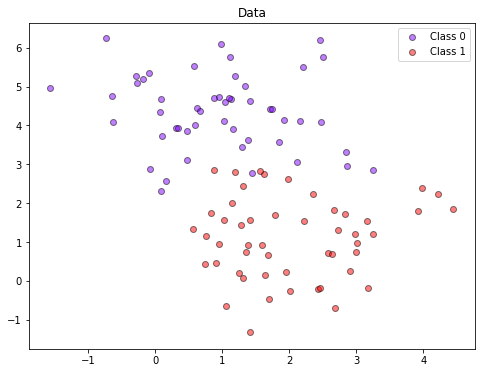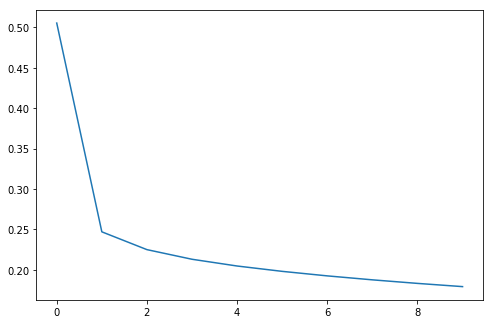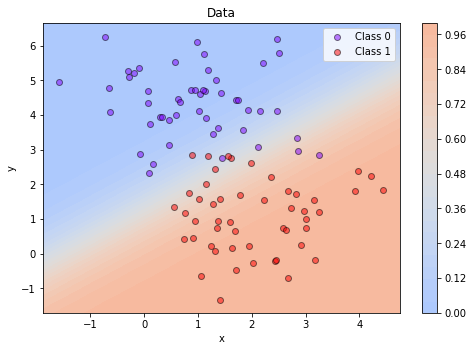# 13 - Introduction to TensorFlow¶

by Fabio A. González, Universidad Nacional de Colombia

version 1.0, June 2018

## Part of the class Applied Deep Learning¶

In :
import tensorflow as tf
from IPython.display import clear_output, Image, display, HTML
import numpy as np
import pylab as pl
from sklearn.datasets.samples_generator import make_blobs

%matplotlib inline

# Helper functions to inline visualization of computing graphs
# Extracted from:
# https://github.com/tensorflow/tensorflow/blob/master/tensorflow/examples/tutorials/deepdream/deepdream.ipynb
def strip_consts(graph_def, max_const_size=32):
"""Strip large constant values from graph_def."""
strip_def = tf.GraphDef()
for n0 in graph_def.node:
n.MergeFrom(n0)
if n.op == 'Const':
tensor = n.attr['value'].tensor
size = len(tensor.tensor_content)
if size > max_const_size:
tensor.tensor_content = "<stripped %d bytes>"%size
return strip_def

def show_graph(graph_def, max_const_size=32):
"""Visualize TensorFlow graph."""
if hasattr(graph_def, 'as_graph_def'):
graph_def = graph_def.as_graph_def()
strip_def = strip_consts(graph_def, max_const_size=max_const_size)
code = """
<script>
document.getElementById("{id}").pbtxt = {data};
}}
</script>
<div style="height:600px">
<tf-graph-basic id="{id}"></tf-graph-basic>
</div>
""".format(data=repr(str(strip_def)), id='graph'+str(np.random.rand()))

iframe = """
<iframe seamless style="width:1200px;height:620px;border:0" srcdoc="{}"></iframe>
""".format(code.replace('"', '&quot;'))
display(HTML(iframe))

# Functions for plotting 2D data and decision regions

def plot_data(X, y):
y_unique = np.unique(y)
colors = pl.cm.rainbow(np.linspace(0.0, 1.0, y_unique.size))
for this_y, color in zip(y_unique, colors):
this_X = X[y == this_y]
pl.scatter(this_X[:, 0], this_X[:, 1],  c=color,
alpha=0.5, edgecolor='k',
label="Class %s" % this_y)
pl.legend(loc="best")
pl.title("Data")

def plot_decision_region(X, pred_fun):
min_x = np.min(X[:, 0])
max_x = np.max(X[:, 0])
min_y = np.min(X[:, 1])
max_y = np.max(X[:, 1])
min_x = min_x - (max_x - min_x) * 0.05
max_x = max_x + (max_x - min_x) * 0.05
min_y = min_y - (max_y - min_y) * 0.05
max_y = max_y + (max_y - min_y) * 0.05
x_vals = np.linspace(min_x, max_x, 30)
y_vals = np.linspace(min_y, max_y, 30)
XX, YY = np.meshgrid(x_vals, y_vals)
grid_r, grid_c = XX.shape
ZZ = np.zeros((grid_r, grid_c))
for i in range(grid_r):
for j in range(grid_c):
ZZ[i, j] = pred_fun(XX[i, j], YY[i, j])
pl.contourf(XX, YY, ZZ, 30, cmap = pl.cm.coolwarm, vmin= -1, vmax=2)
pl.colorbar()
pl.xlabel("x")
pl.ylabel("y")

/Users/fgonza/anaconda3/envs/TF/lib/python3.6/site-packages/h5py/__init__.py:36: FutureWarning: Conversion of the second argument of issubdtype from float to np.floating is deprecated. In future, it will be treated as np.float64 == np.dtype(float).type.
from ._conv import register_converters as _register_converters


### 1. TensorFlow basics¶

First we will create a simple computing graph and visualize it. The visualization is interactive, so you can expand and collapse nodes, zoom in, zoom out, pan, etc.

In :
graph = tf.Graph()

with graph.as_default():
a = tf.constant(10, tf.float32, name= 'a')
b = tf.constant(-5, tf.float32, name= 'b')
c = tf.constant(4, tf.float32, name= 'c')

x = tf.placeholder(tf.float32, name= 'x')
new_exp = x + c
y = a * x * x + b * x + c

show_graph(graph.as_graph_def())


If we try to evaluate y it produces an object, not a value:

In :
y

Out:
<tf.Tensor 'add_2:0' shape=<unknown> dtype=float32>

To evaluate the expression we have to give a value to create a session and run the graph giving a value to x:

In :
with graph.as_default():
sess = tf.Session()
result = sess.run(new_exp, {x: 5.0})
sess.close()

print('y =', result)

y = 9.0


We can calculate the gradient of y with respect to a variable (or placeholder) in the graph:

In :
with graph.as_default():

sess = tf.Session()
result = sess.run(y_prime, {x: 5.0})
sess.close()

In :
print(result)

[95.0]


This expands the graph with the gradient calculation component:

In :
show_graph(graph.as_graph_def())


We can use gradient descent to find a minimum of the polynomial represented in the graph. Here we need to change x to be a variable because its value is going to be updated for different sucessive evaluations of the graph.

In :
graph = tf.Graph()
with graph.as_default():
a = tf.constant(10, tf.float32, name= 'a')
b = tf.constant(-5, tf.float32, name= 'b')
c = tf.constant(4, tf.float32, name= 'c')
x = tf.Variable(0.0, name= 'x')
y = a * x * x + b * x + c

update = optimizer.minimize(y)

# Graph execution
sess = tf.Session()
sess.run(tf.global_variables_initializer())
for i in range(20):
val_y, val_x, _ = sess.run([y, x, update])
print(i, val_x, val_y)
sess.close()

0 0.099999994 4.0
1 0.16 3.6
2 0.196 3.456
3 0.21759999 3.446496
4 0.23055999 3.3854978
5 0.238336 3.378779
6 0.2430016 3.3763604
7 0.24580096 3.3754897
8 0.24748057 3.3751762
9 0.24848834 3.3750634
10 0.24909301 3.375023
11 0.24945581 3.375008
12 0.24967349 3.3744578
13 0.2498041 3.3750012
14 0.24988246 3.3750002
15 0.24992947 3.375
16 0.24995768 3.375
17 0.24997461 3.375
18 0.24998477 3.375
19 0.24999087 3.375


Again the graph is expanded to contain the gradient calculations and the update rules of the optimizer. You can see two new containers gradients and GradientDescent which can be expanded.

In :
show_graph(graph.as_graph_def())


### 2. Neural network training¶

Now we will implement a logistic regression model to classify the following data:

In :
X, Y = make_blobs(n_samples=100, centers=2, n_features=2, random_state=0)
pl.figure(figsize=(8, 6))
plot_data(X, Y)The following graph implements a logistic regression model. Scopes help to organize the graph in submodules. x and y_true are the main inputs to the model. None in the shape indicates that they can fit inputs with different number of samples, so we can present the whole training dataset or minibatches. The inference module corresponds to the prediction part of the module. Notice that y_pred is just the lineal combination, it does not apply the logistic function. This is done directly in the loss function. The loss module specify the loss function to use, in this case, sigmoid_cross_entropy_with_logits the logits correspond to the linear combination in y_pred. The reduce tf.reduce_mean function calculates a mean loss for all the samples that were presented at once. The train module includes a gradien descent optimizer.

In :
graph = tf.Graph()
with graph.as_default():
x = tf.placeholder(tf.float32,shape=[None,2])
y_true = tf.placeholder(tf.float32,shape=None)

with tf.name_scope('inference') as scope:
w = tf.Variable([[0,0]],dtype=tf.float32,name='weights')
b = tf.Variable(0,dtype=tf.float32,name='bias')
y_pred = tf.matmul(w,tf.transpose(x)) + b

with tf.name_scope('loss') as scope:
loss = tf.nn.sigmoid_cross_entropy_with_logits(labels=y_true,logits=y_pred)
loss = tf.reduce_mean(loss)

with tf.name_scope('train') as scope:
learning_rate = 1.0
train = optimizer.minimize(loss)

init = tf.global_variables_initializer()

show_graph(graph.as_graph_def())


The optimization algorithm is run for num_epochs iterations or epochs. In each epoch the whole training dataset is presented. Each five iterations, we run the model to calculate the loss, which is stored and later plotted. Notice that the session is not closed since we will need the values of the variables to predict additional samples.

In :
num_epochs = 50
losses = []

with graph.as_default():
sess = tf.Session()
sess.run(init)
for step in range(num_epochs):
sess.run(train,{x: X, y_true: Y})
if (step % 5 == 0):
losses.append(sess.run(loss, {x: X, y_true: Y}))

pl.figure(figsize = (8,16/3))
pl.plot(losses)

Out:
[<matplotlib.lines.Line2D at 0x1a1c5153c8>]Now we use the plot_decision_function, defined at the beginning of the handout, to visualize the decision region. Notice the that the graph is run as many times as different values the visualization grid has, this is very inefficient! The efficient way to do it is passing all the values at the same time to the graph. However, for this example the inefficient method works just fine.

In :
def sigmoid(x):
return 1.0/(1.0 + np.exp(-x))

with graph.as_default():
wval = sess.run(w)
print(wval)
result = sess.run(y_pred, {x:np.array([[1,2]])})
print(result)
def pred_fun(x1, x2):
xval = np.array([[x1, x2]])
return sigmoid(sess.run(y_pred,{x: xval}))

pl.figure(figsize = (8,16/3))
plot_decision_region(X, pred_fun)
plot_data(X, Y)

[[ 1.3167489 -1.5882863]]
[[0.07089913]]Clossing the session.

In :
 sess.close()


### 3. Using TensorBoard¶

TensorBoard is an application provided by TensorFlow that allows to monitor the training process. TensorBoard uses as input log files which are created by a summary mechanism. Summaries correspond to operators in the graph that calculate statistics of other graph variables.

The following function calculates different summary statistics for a given variable:

In :
def variable_summaries(var):
with tf.name_scope('summaries'):
mean = tf.reduce_mean(var)
tf.summary.scalar('mean', mean)
with tf.name_scope('stddev'):
stddev = tf.sqrt(tf.reduce_mean(tf.square(var - mean)))
tf.summary.scalar('stddev', stddev)
tf.summary.scalar('max', tf.reduce_max(var))
tf.summary.scalar('min', tf.reduce_min(var))
tf.summary.histogram('histogram', var)


We define a new graph that includes these summaries:

In :
graph = tf.Graph()
with graph.as_default():
x = tf.placeholder(tf.float32,shape=[None,2])
y_true = tf.placeholder(tf.float32,shape=None)

with tf.name_scope('inference') as scope:
w = tf.Variable([[0,0]],dtype=tf.float32,name='weights')
b = tf.Variable(0,dtype=tf.float32,name='bias')
y_pred = tf.matmul(w,tf.transpose(x)) + b
variable_summaries(w)
variable_summaries(b)

with tf.name_scope('loss') as scope:
loss = tf.nn.sigmoid_cross_entropy_with_logits(labels=y_true,logits=y_pred)
loss = tf.reduce_mean(loss)
variable_summaries(loss)

with tf.name_scope('train') as scope:
learning_rate = 1.0
train = optimizer.minimize(loss)

merged = tf.summary.merge_all()
init = tf.global_variables_initializer()

show_graph(graph.as_graph_def())


We will split the dataset in train and test, so that we can compute both the training and validation loss. We create a train_writer and a test_writer that that work as handles to write the respective logs.

In :
x_train = X[:50]
x_test  = X[50:]
y_train = Y[:50]
y_test  = Y[50:]

LOG_DIR = 'logs'
train_writer = tf.summary.FileWriter(LOG_DIR + '/train',
graph=graph)
test_writer = tf.summary.FileWriter(LOG_DIR + '/test')

num_epochs = 400

with graph.as_default():
with tf.Session() as sess:
sess.run(init)
for step in range(num_epochs):
summary, train_loss, _ = sess.run([merged, loss, train] ,{x: x_train, y_true: y_train})
summary, val_loss = sess.run([merged, loss] ,{x: x_test, y_true: y_test})
if step % 20 == 0:
print(step, train_loss, val_loss)


0 0.6931472 0.43206224
20 0.16180742 0.27600694
40 0.14276873 0.27437234
60 0.13373153 0.27041867
80 0.12786473 0.2650913
100 0.123512484 0.2591867
120 0.12005596 0.25315437
140 0.11720215 0.24724531
160 0.11478849 0.24159819
180 0.112713665 0.23628533
200 0.110909075 0.23133859
220 0.1093251 0.22676502
240 0.10792431 0.22255598
260 0.10667751 0.218694
280 0.105561465 0.21515647
300 0.104557216 0.21191847
320 0.10364933 0.20895454
340 0.10282493 0.20623972
360 0.1020733 0.20375048
380 0.101385444 0.20146467


Finally, we can launch TensorBoard from the console wiht the following command:

tensorboard --logdir=logs/

After launching it, we need to visit: http://localhost:6006 or whatever address TensroBoard tells us.

In [ ]: